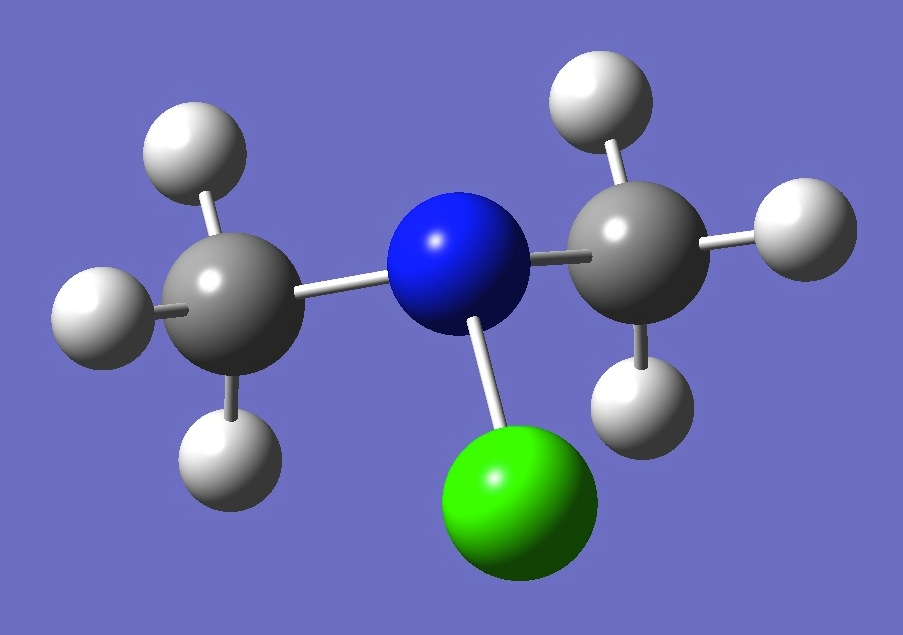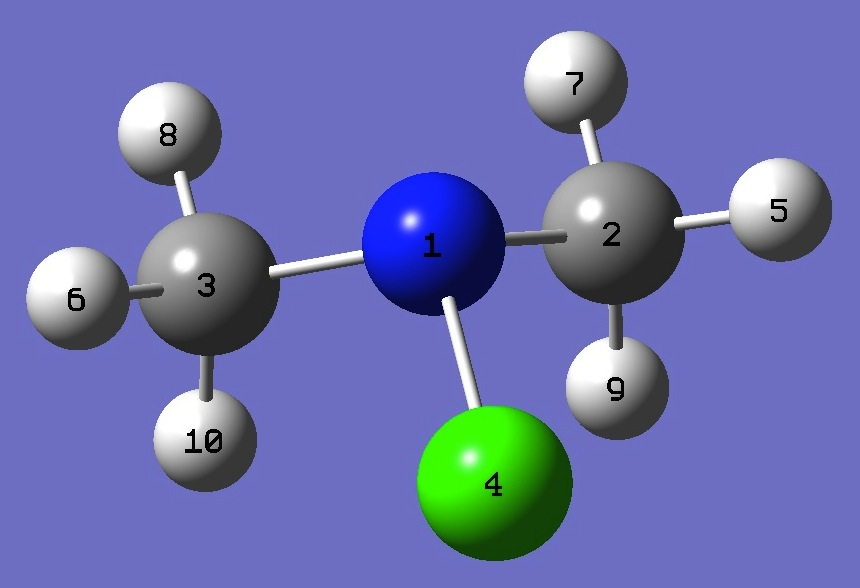(CH3)2NClChlorine and Nitrogen

Nuclear Quadrupole Coupling Constants

in Dimethylchloroamine

Cl nqcc's in (CH3)2NCl were determined by Durig et al. .

Calculation of the chlorine - and nitrogen - nqcc tensors was made here on ropt structures given by MP2/6-311+G(3df,3pd) and MP2/aug-cc-pVTZ optimization. These are compared with the experimental chlorine nqcc's in Table 1.  Calculated nitrogen nqcc's are given in Table 2.  Structure parameters in Z-matrix format are given in Table 3; rotational constants in Table 4.

In Tables 1 and 2, subscripts a,b,c refer to the principal axes of the inertia tensor, subscripts x,y,z to the principal axes of the nqcc tensor.  Ø (degrees) is the angle between its subscripted parameters. ETA = (Xxx - Xyy)/Xzz.

RMS is the root mean square difference between calculated and experimental nqcc's (percentage of the average experimental nqcc).  RSD is the residual standard deviation of calibration of the model for calculation of the efg's/nqcc's (see Chlorine, Nitrogen).

 Table 1.  Cl nqcc's in (CH3)2NCl (MHz).  Calculation was made on (1) MP2/6-311+G(3df,3pd) and (2) MP2/aug-cc-pVTZ optimized structures. Calc (1) Calc (2) Expt.  Xaa (35Cl) - 82.47 - 82.24 - 81.86 Xbb 46.18 46.18 45.75 Xcc 36.29 36.06 36.11 |Xac| 36.90 37.21 RMS 0.45 (0.8 %) 0.33 (0.6 %) RSD 0.49 (1.1 %) 0.49 (1.1 %) Xxx 46.83 46.79 Xyy 46.18 46.18 Xzz - 93.01 - 92.97 ETA - 0.0069 - 0.0066 Øz,a 15.93 16.09 Øa,NCl 15.84 15.94 Øz,NCl 0.09 0.14 Xaa (37Cl) - 65.06 - 64.88 - 64.58 Xbb 36.39 36.39 36.06 Xcc 28.67 28.49 28.52 |Xac| 28.98 29.22 RMS 0.35 (0.8 %) 0.26 (0.6 %) RSD 0.44 (1.1 %) 0.44 (1.1 %)

 Table 2.  14N nqcc's in (CH3)2N35Cl (MHz).  Calculation was made on (1) MP2/6-311+G(3df,3pd) and (2) MP2/aug-cc-pVTZ optimized structures. Calc (1) Calc (2) Expt. Xaa 5.386 5.408 Xbb 1.015 0.957 Xcc - 6.401 - 6.365 |Xac| 1.518 1.569 RSD 0.030 (1.3 %) 0.030 (1.3 %) Xxx 5.578 5.613 Xyy 1.015 0.957 Xzz - 6.593 - 6.570 ETA - 0.692 - 0.709 Øz,a 82.78 82.54 Øa,NCl 15.84 15.94 Øz,NCl 98.62 98.38

 Table 3.  (CH3)2NCl  Structure parameters (Å and degrees).  ropt(1) = MP2/6-311+G(3df,3pd) and ropt(2) = MP2/aug-cc-pVTZ optimized structures.N  C,1,B1  C,1,B1,2,A1  Cl,1,B3,3,A2,2,D1,0  H,2,B4,1,A3,3,D2,0  H,3,B4,1,A3,2,-D2,0  H,2,B6,1,A5,3,D4,0  H,3,B6,1,A5,2,-D4,0  H,2,B8,1,A7,3,D6,0  H,3,B8,1,A7,2,-D6,0 ropt(1) ropt(2) B1=1.45912403  B3=1.73951558  B4=1.08589362  B6=1.08900071  B8=1.09593626  A1=110.5831514  A2=107.61975208  A3=109.32528053  A5=107.16307364  A7=112.37746748  D1=-117.29202905  D2=-178.30361883  D4=63.63356621  D6=-56.61366534 B1=1.46072979  B3=1.75093036  B4=1.08674957  B6=1.0899983  B8=1.09645101  A1=110.45478334  A2=107.26987329  A3=109.30155946  A5=107.01033826  A7=112.40481378  D1=-116.60048708  D2=-177.77419629  D4=64.11884383  D6=-56.01441634

 Table 4.  (CH3)2N35Cl  Rotational constants (MHz):  ropt(1) = MP2/6-311+G(3df,3pd) and ropt(2) = MP2/aug-cc-pVTZ optimized structures. ropt(1) ropt(2) Expt.  A 9116. 9097. 8992.50(29) B 5036. 5006. 4967.38(2) C 3528. 3515. 3473.52(1)

 J.R.Durig, K.K.Chatterjee, N.E.Lindsay, and P.Groner, J.Am.Chem.Soc. 108,6903(1986).

CH3NH2 CH3NHCl NH2Cl (CH3)2NH

Table of Contents

Molecules/Chlorine

Molecules/Nitrogen

CH32NCl.html

Last Modified 8 May 2016